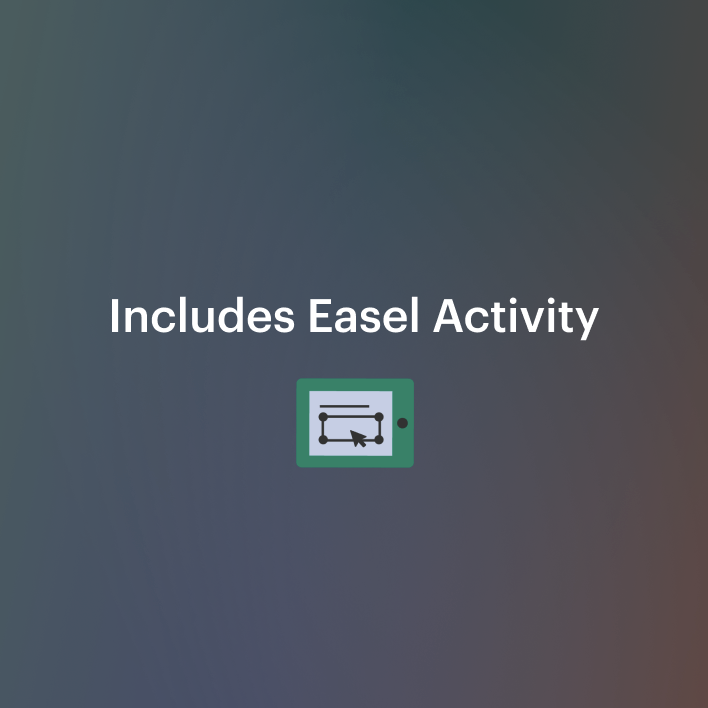# Converting Customary Units of Measurement Activity - Jack and the Beanstalk4th - 5th, Homeschool
Subjects
Standards
Resource Type
Formats Included
• PDF
•Activity
Pages
35 pages
Easel Activity Included
This resource includes a ready-to-use interactive activity students can complete on any device. Easel by TpT is free to use! Learn more.

#### Also included in

1. Kids master equivalencies and have fun with this complete customary measurement unit. Learning scaffolds from simple problems with conversion tables to word problems. Then they extend understanding with mixed measures and mixed measures word problems. Before the assessment, kids practice with the "J
\$9.00
\$11.00
Save \$2.00

### Description

Measurement is woven into this version of Jack and the Beanstalk. Fourth and fifth grade students solve math word problems involving elapsed time and customary unit conversions. They add, subtract, multiply, divide, compare, and solve multi-step problems.

You can now create an Easel Activity for this resource. The files have been customized for you and are ready to share.

What's Included:

• Lesson plans
• Directions for sharing the digital version through Google Classroom
• Best practices
• Hook
• 16-page math activity books featuring Jack and the Beanstalk.
• Two versions parallel versions for differentiation - one more difficult and one less difficult with conversions listed at the bottom of the page
• Foldable or full-page options
• Five conversion tables

Exercises:

• Multiply to find out how many pints Jack's cow gives each week then convert to quarts and gallons
• Calculate elapsed time to see if he will arrive at the market on time
• Convert inches to yards to see if he has enough rope
• Calculate elapsed time to see how long he has to wait for breakfast
• Subtract mixed measures to see how much the beanstalk grew
• Change pounds and ounces to determine how many trips Jack needs to make
• Calculate elapsed time to find out how long he has to eat his breakfast
• Exchange pounds for ounces then multiply to find out how much the gold is worth
• Convert gallons to cups to determine how much punch was in the vat
• Change pounds to ounces then multiply to find out how much he could earn each week from the hen's golden eggs
• Calculate elapsed time to find out how much time Jack has to study for his measurement test

Enjoy Teaching!

Brenda Kovich

Would you like to know when I post more resources? Simply follow me on TpT.

I'm committed to continual improvement. This resource was updated and enhanced on May 24, 2020.

Total Pages
35 pages
Included
Teaching Duration
1 hour
Report this Resource to TpT
Reported resources will be reviewed by our team. Report this resource to let us know if this resource violates TpT’s content guidelines.

### Standards

to see state-specific standards (only available in the US).
Convert among different-sized standard measurement units within a given measurement system (e.g., convert 5 cm to 0.05 m), and use these conversions in solving multi-step, real world problems.
Use the four operations to solve word problems involving distances, intervals of time, liquid volumes, masses of objects, and money, including problems involving simple fractions or decimals, and problems that require expressing measurements given in a larger unit in terms of a smaller unit. Represent measurement quantities using diagrams such as number line diagrams that feature a measurement scale.
Know relative sizes of measurement units within one system of units including km, m, cm; kg, g; lb, oz.; l, ml; hr, min, sec. Within a single system of measurement, express measurements in a larger unit in terms of a smaller unit. Record measurement equivalents in a two-column table. For example, know that 1 ft is 12 times as long as 1 in. Express the length of a 4 ft snake as 48 in. Generate a conversion table for feet and inches listing the number pairs (1, 12), (2, 24), (3, 36),...